# R facet_grid() Function

The facet_grid() function is used when you want to create a matrix of panels defined by rows and columns.

The facet grids are a great way to visualize data across multiple subplots based on different levels of one or more categorical variables. It’s a simple way to break down a dataset into multiple views based on specific categories.

For this project, a dataset with multiple categorical variables and at least one continuous variable would be ideal.

## Syntax

``````facet_grid(
rows = NULL,
cols = NULL,
scales = "fixed",
space = "fixed",
shrink = TRUE,
labeller = "label_value",
as.table = TRUE,
switch = NULL,
drop = TRUE,
margins = FALSE,
facets = deprecated()
)``````

## Creating facet grids

Here is the step-by-step guide:

### Step 1: Install and load the necessary libraries

``````install.packages("tidyverse")

install.packages("ggplot2")``````

``````library(tidyverse)
library(ggplot2)``````

### Step 2: Load the dataset into a data frame

To get an overview of the dataset, use the read_csv() built-in function to import the dataset into a data frame and then use the head() function.

``````library(tidyverse)
library(ggplot2)

``````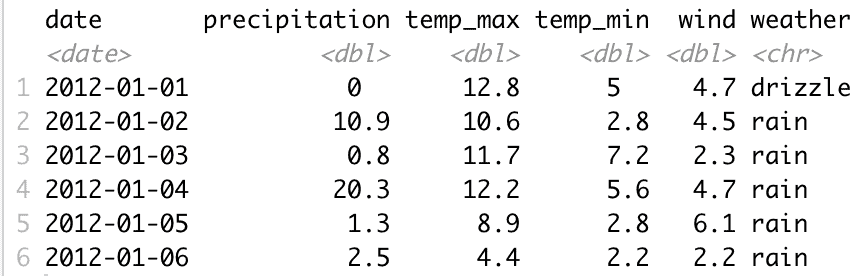### Step 3: Data Visualization

Based on our dataset, facet_grid() might be a good use of a facet by year and weather type.

We must extract the year from the date column and use it in the function.

``````# Extract the year from the date column
weather_data\$year <- as.integer(format(as.Date(weather_data\$date), "%Y"))
``````

Now, you can create the plots.

``````library(tidyverse)
library(ggplot2)

# Extract the year from the date column
weather_data\$year <- as.integer(format(as.Date(weather_data\$date), "%Y"))

# Use facet_grid to plot
ggplot(weather_data, aes(x = date, y = temp_max)) +
geom_line(aes(color = "Max Temp"), na.rm = TRUE) +
geom_line(aes(y = temp_min, color = "Min Temp"), na.rm = TRUE) +
facet_grid(year ~ weather, scales = "free_y") +
labs(title = "Temperature Trends by Year and Weather Type", x = "Date",
y = "Temperature (°C)", color = "Legend") +
theme_minimal()``````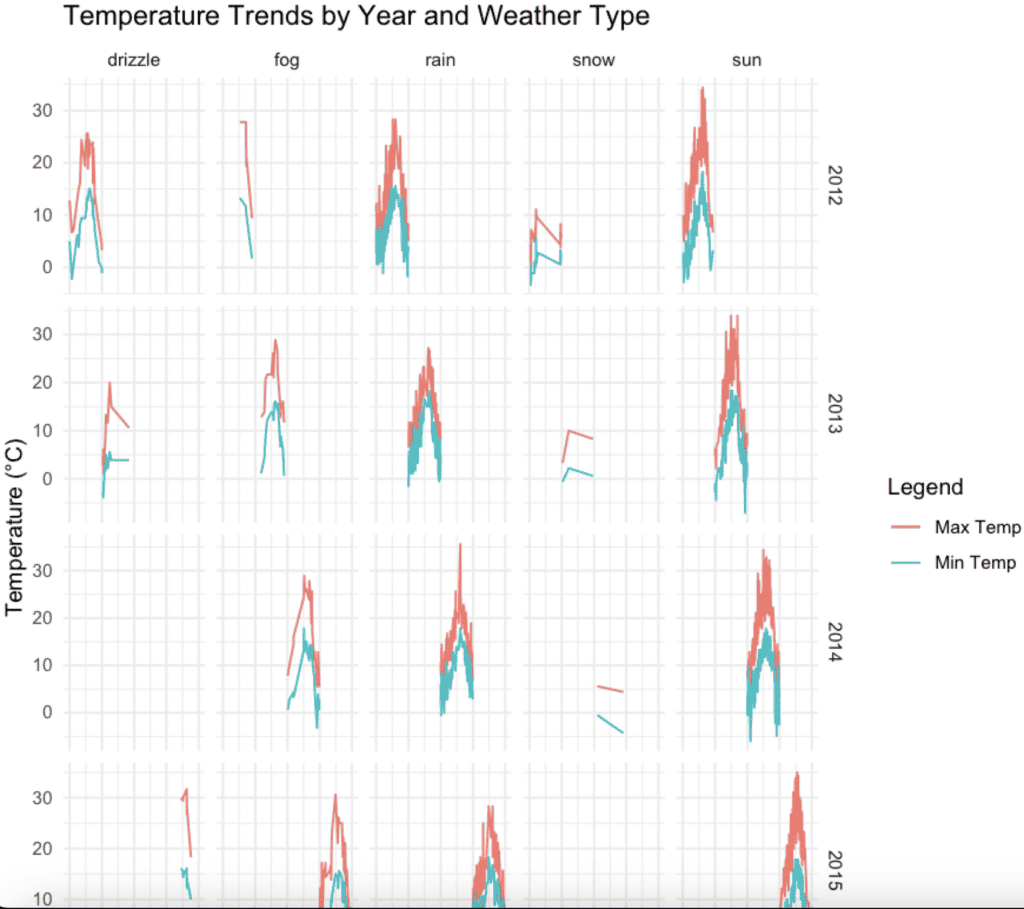We get a grid of plots where each row represents a year, and each column represents a weather type, showing temperature trends for each combination.

## Facet by Month and Weather

Another interesting visualization might be to see how the weather patterns and temperatures change by month and by weather type.

To do this, we will first need to extract the month from the date column:

``````# Extract the month from the date column
weather_data\$month <- as.integer(format(as.Date(weather_data\$date), "%m"))

# Use facet_grid to plot
ggplot(weather_data, aes(x = date, y = temp_max)) +
geom_line(aes(color = "Max Temp"), na.rm = TRUE) +
geom_line(aes(y = temp_min, color = "Min Temp"), na.rm = TRUE) +
facet_grid(month ~ weather, scales = "free_y") +
labs(title = "Temperature Trends by Month and Weather Type",
x = "Date", y = "Temperature (°C)", color = "Legend") +
theme_minimal()``````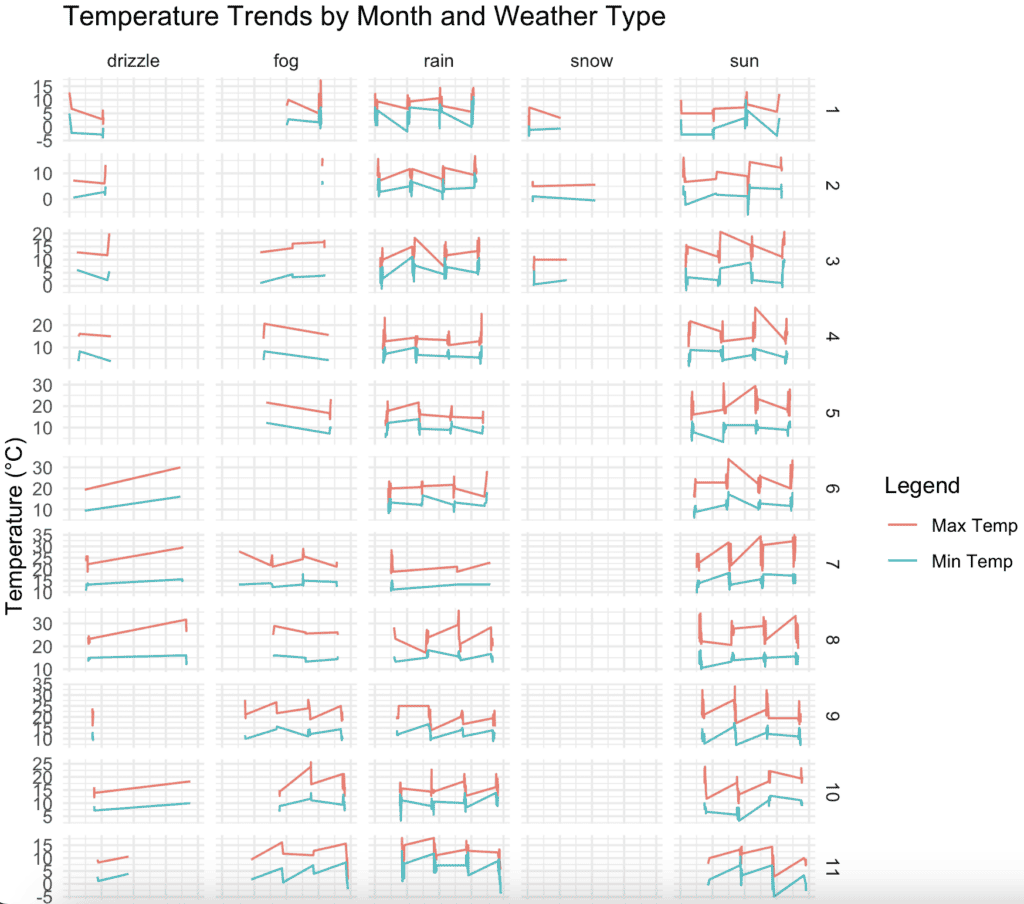## Facet by Year and Month

We will see how temperatures have varied over the years for each month:

When using facet_grid(year ~ month), we should ensure that year and month are included in the main aes() call.

``````library(tidyverse)
library(ggplot2)

# Extract the year and month from the date column
weather_data\$year <- as.integer(format(as.Date(weather_data\$date), "%Y"))
weather_data\$month <- as.integer(format(as.Date(weather_data\$date), "%m"))

# Use facet_grid to plot
ggplot(weather_data, aes(x = date, y = temp_max)) +
geom_line(aes(color = "Max Temp"), na.rm = TRUE) +
geom_line(aes(y = temp_min, color = "Min Temp"), na.rm = TRUE) +
facet_grid(year ~ month, scales = "free_y") +
labs(title = "Temperature Trends by Year and Month",
x = "Date", y = "Temperature (°C)", color = "Legend") +
theme_minimal()``````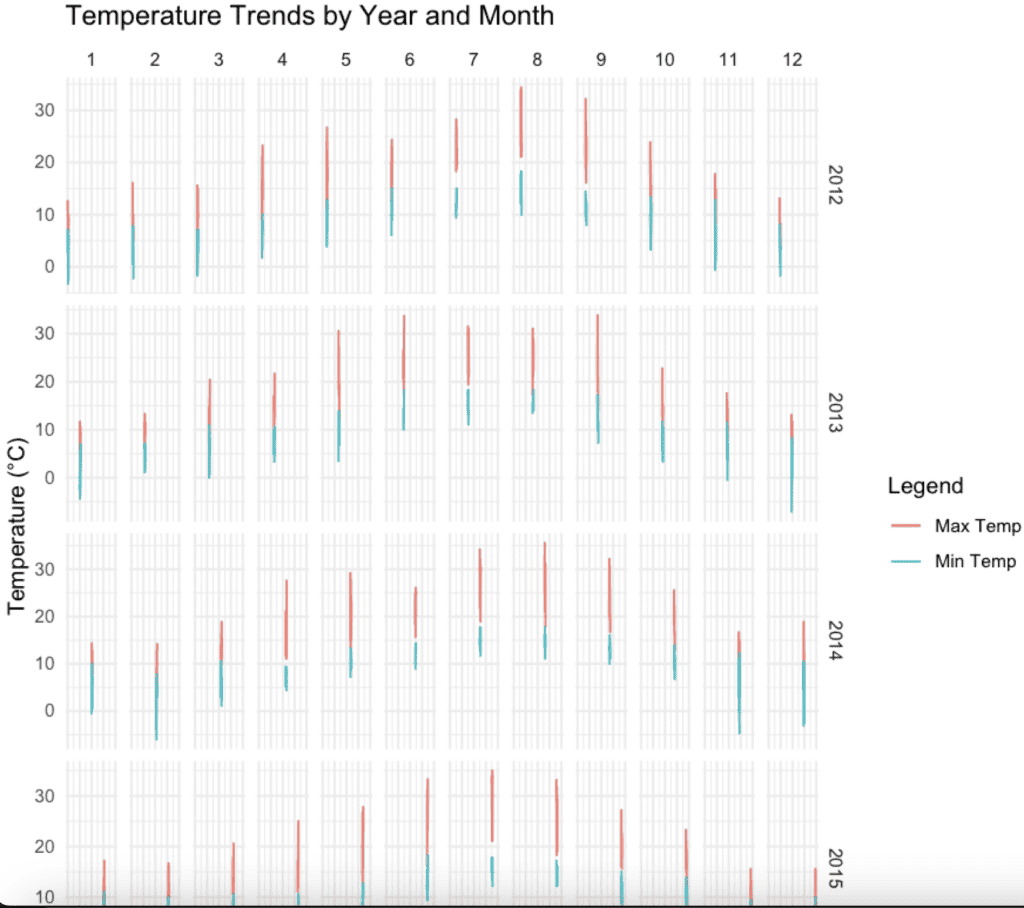## Facet by Wind Speed Categories and Weather

We can categorize wind speed into different levels (e.g., Low, Medium, High) and then facet by these categories:

``````library(tidyverse)
library(ggplot2)

# Create wind speed categories
weather_data\$wind_category <- cut(weather_data\$wind,
breaks = c(0, 3, 6, Inf),
labels = c("Low", "Medium", "High"))

# Use facet_grid to plot
ggplot(weather_data, aes(x = date, y = temp_max)) +
geom_line(aes(color = "Max Temp"), na.rm = TRUE) +
geom_line(aes(y = temp_min, color = "Min Temp"), na.rm = TRUE) +
facet_grid(wind_category ~ weather, scales = "free_y") +
labs(title = "Temperature Trends by Wind Speed and Weather Type",
x = "Date", y = "Temperature (°C)", color = "Legend") +
theme_minimal()``````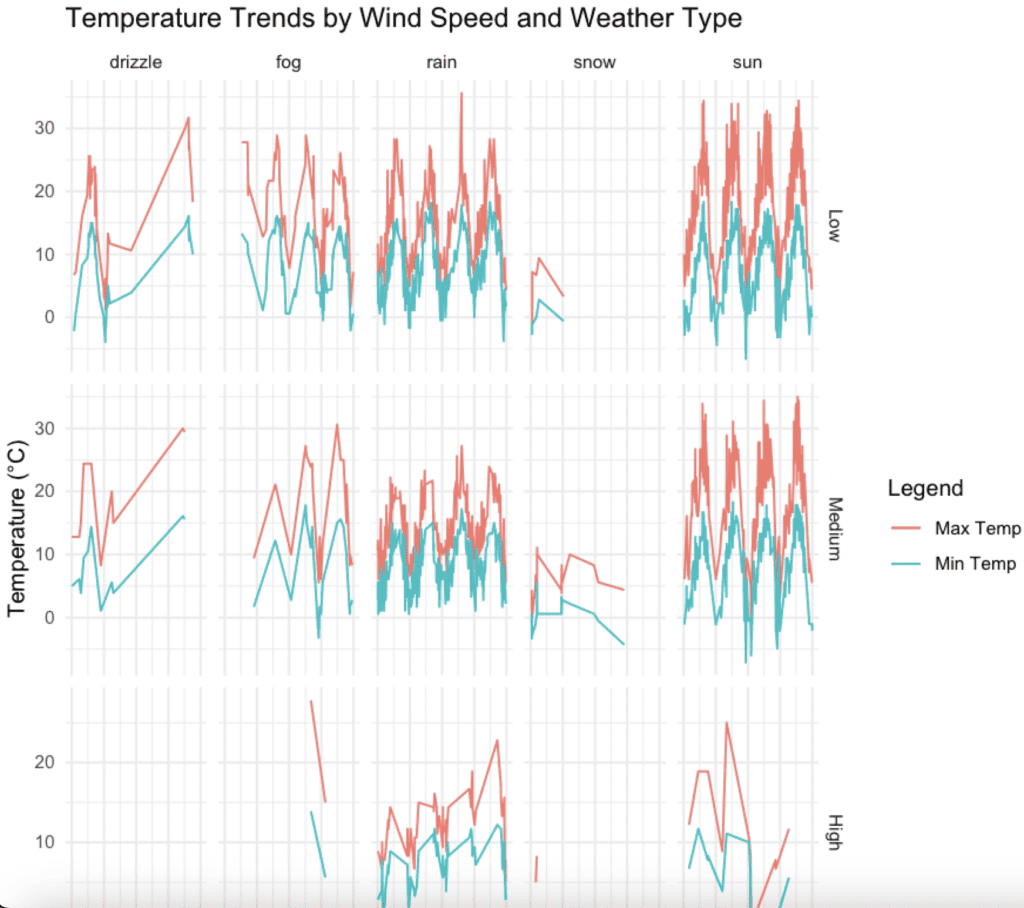That’s all!